Art Deco Cameras

# Finding the focal length of a vintage camera

## Why you might need to know the focal length

Many vintage cameras do not document the focal length. However, if you want to use them successfully with modern film, you may need to know the focal length. The focal length will be needed to:-

• Calculate the f number of the aperture
• Use a depth of field calculator
• Calculate angle of view of the camera

## Direct measurement of single lens

If the camera uses a single lens then direct measurement is possible. The single lens could be a convex lens or a meniscus lens. Simply measure the distance between the film plane and the edge of the lens when the lens is focussed on infinity. This is the focal length. If the focus is fixed, it is normally focussed at the hyperfocal distance. Kodak fixed focus cameras are focussed at 25ft (7.6m). This adds about 1.5% to the distance between the lens and the film plane for a 120, 620 116 or 616 camera and about 1% for a 127 camera. These amounts can be subtraced to get a better accuracy.

However, there are things to look out for. Some cameras have a plain glass cover at the front of the camera. Examples are the Paris Gap 6x9, the Kodak Brownie Target Six-20, the Kodak Six-20 Brownie Junior and the Kodak Six-16 Brownie Junior. In these cases you must measure to the meniscus lens inside the camera. A cotton bud taped to the end of a straw might make a suitable measuring stick so you don't damage the lens. In some cases you will need to put the camera in 'T' mode to keep the shutter open and measure through the aperture and shutter.

To determine what type of lens you have, look at the reflection of a bright object in the lens. Move the lens around a bit. If you see a single reflection, it's probably plain glass. If you see two reflections, one smaller than the other it will be a meniscus lens. If you see two reflections with one inverted, it will be a bi-convex lens.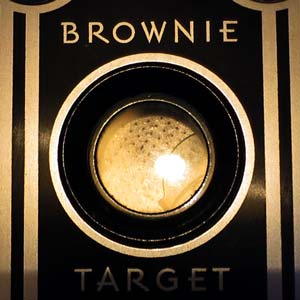Plain Glass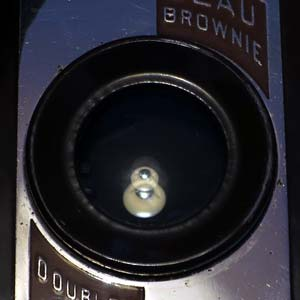MeniscusBi-Convex

## Multi-lens Systems

### Approximation

The focal length of a duplex or triplet lens is more difficult to measure as you cannot simply measure to one of the lenses. The focal length will be somewhere between the two or three lenses. An approximate focal length can be found by taking the distance from the film plane to halfway between the front and back lens. For example, lets estimate the focal length of a Kodak Beau Brownie No. 2A. The distance from the focal plane to the front lens is 125mm and to the back lens is 100mm. The focal length will be somewhere between the two , say 112mm. However, if you look at the reflections in the front and back lenses, you will notice that the difference in the size of the two reflections in the rear lens is bigger that that of the front lens. So, the back lens is probably stronger than the front lens. So the principal focus will be nearer the back lens - say 105mm. Don't expect too much accuracy here.

This does not work for more complex lenses because the effective focal length could be outside the lens limits.

As indicated above, some cameras have plain glass at the front so these must be ignored and the measurement is from the meniscus lens at the back.

## Experimental Procedure

This experimental procedure will yield much better accuracy and will work for all lens types.

Assuming that the principal axis of the lens system is at 'P' and the object size is 'Y' and the image size is 'X' and the focal length of the simple or compound system is f. Also assume that the object Y is at sufficient distance such that the image is in focus on the image plane. Z is the distance between the object and the image plane.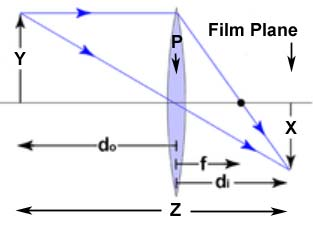Ray Diagram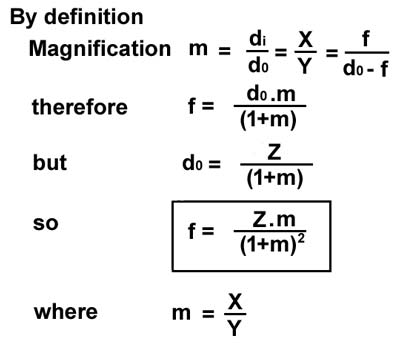### General Method

So the upshot of this is to focus on a large object and to measure the object size(Y), the image size(X) and the distance from the object to the film plane(Z). Apply the formula.

Remember to use the same units throughout. Best to work in millimetres(mm) ie. 2cm = 20mm; 2m=2000mm### Method 1

Focus on a large object of known width(Y) at a known distance(Z). Fixed focus cameras are normally focused at the hyperfocal distance so having the object at that distance would be ideal. However if your object is at least 2 - 3 meters away that should give reasonable accuracy. The image of the object should almost fill the film plane. Take a picture. Have the film developed. On the negative, measure the image size(X) of the object. Apply the formula.

### Method 2

Open the back of the camera and tape some translucent material on the film plane. You could use baking paper, greaseproof paper or a piece of ground glass.

Put the camera on a tripod. Place two small bare light sources about 2 meters apart and at a distance of 2-3 meters. Small spotlights facing the camera are ideal. Putting tape on the spots to make two vertical slits is even better. Only tape the spots if LED lamps are used - overheating! With the camera in T or B mode focus on the lights, view the lights on the translucent material.

Measure the distance between the lights(Y), the perpendicular distance from the centre between the lights and the film plane(Z) and the distance between the two spots of light on the translucent material(X).

Apply the formula.

### Example

Let's try method 2 with a Kodak Beau Brownie 2A. See the set up below:-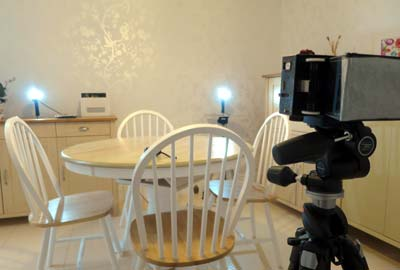Set Up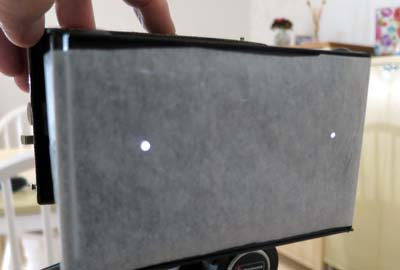Image of Spots

The measured values were:-

• Distance from Spots to image plane Z=2530mm
• Distance spots apart Y=1650mm
• Distance between image spots X=71mm.

Putting these values into the equation above we get f=100.07mm. I'd probably round this to 100mm.

### Handy Calculator

Here is a handy calculator that will do the calculation for you. Make sure you input all measurements in millimetres (mm). The result is rounded to the nearest mm.

 Focal Length Calculator Object to Film Plane (Z) mm Object Size (Y) mm Image Size (X) mm Focal Length mm

### Conclusion

The accuracy of the above methods depend on the accuracy of your measurements and whether the image is properly in focus. The most sensitive to measurement error is the image size. For instance, if the image size is 50mm then a 0.5mm error in measurement will cause a 1% error in the calculation of the focal length. The above method will provide sufficient accuracy of the focal length for the calculation of:- depth of field; aperture number; angle of view; field of view.